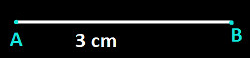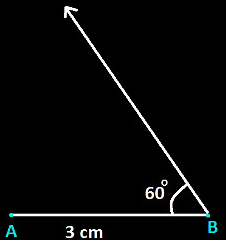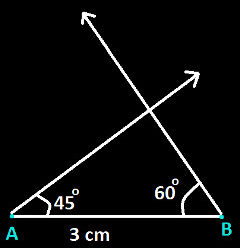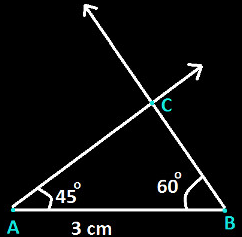# Constructing Triangles, ASA

Constructing ASA triangles explains to construct a triangle where the measure of two angles and one of the lengths of the side is given to us. Construction of similar triangles is easy because we can easily measure the angles and sides with the help of ruler and compass. Only we need to follow the designated steps. Let us learn here how to construct a triangle whose measurement of one side and two angles are given to us.

## ASA Triangle Construction

For the ASA criterion to be satisfied, the given side must necessarily be the one that is enclosed by the known angles. The criterion does not hold if any of the other sides’ measurements is provided.

Now coming back to constructing triangles with the ASA criterion, you will require a ruler and a protractor. Let us say, in a triangle ABC, the measures of the angles are ∠CAB = 45 degrees and ∠ABC = 60 degrees. The length of side AB = 3cm. The steps for its construction are as follows:

• Step 1: Using the ruler, construct a line segment AB of length 3 cm.• Step 2: Using the protractor, draw a ray at point B making 60 degrees with the line BA.• Step 3: Similarly, draw a ray at point A making 45 degrees with the line AB using the protractor.• Step 4: Mark the point where the two rays meet as C.Thus, you obtain a triangle ABC of the required measurements.